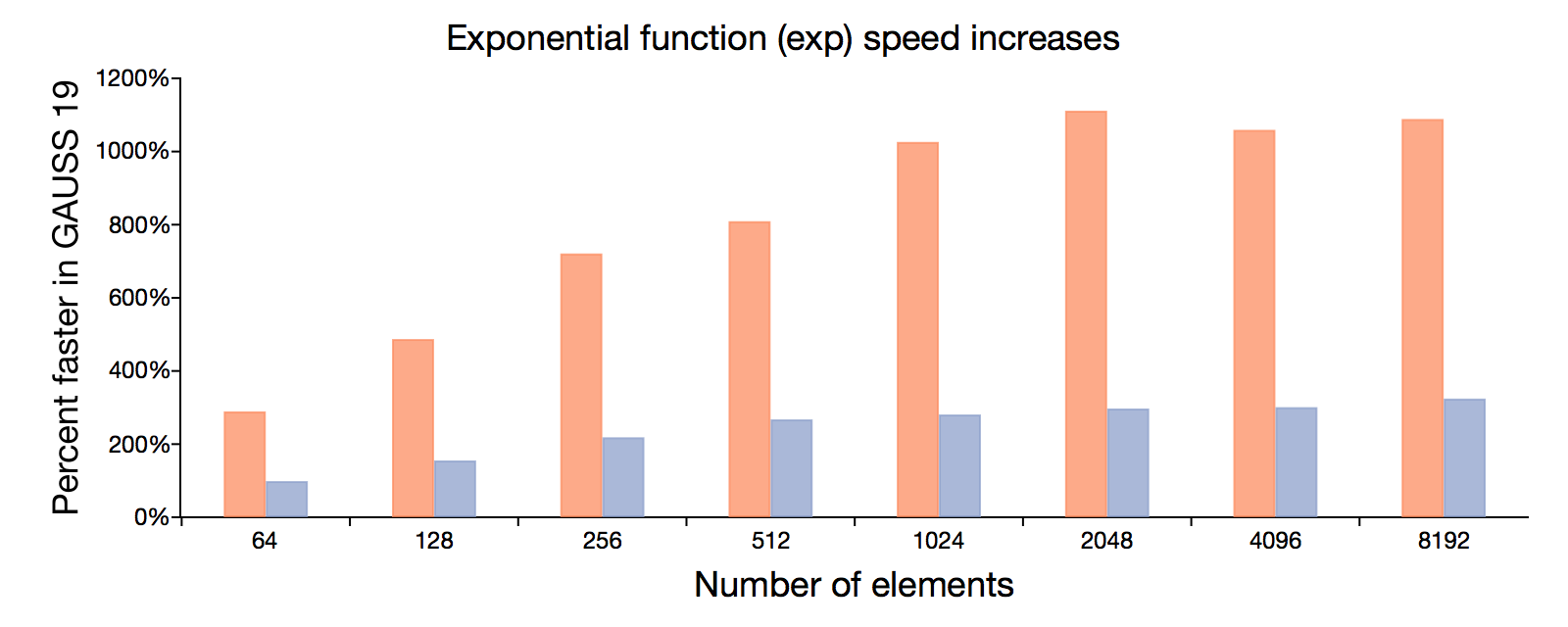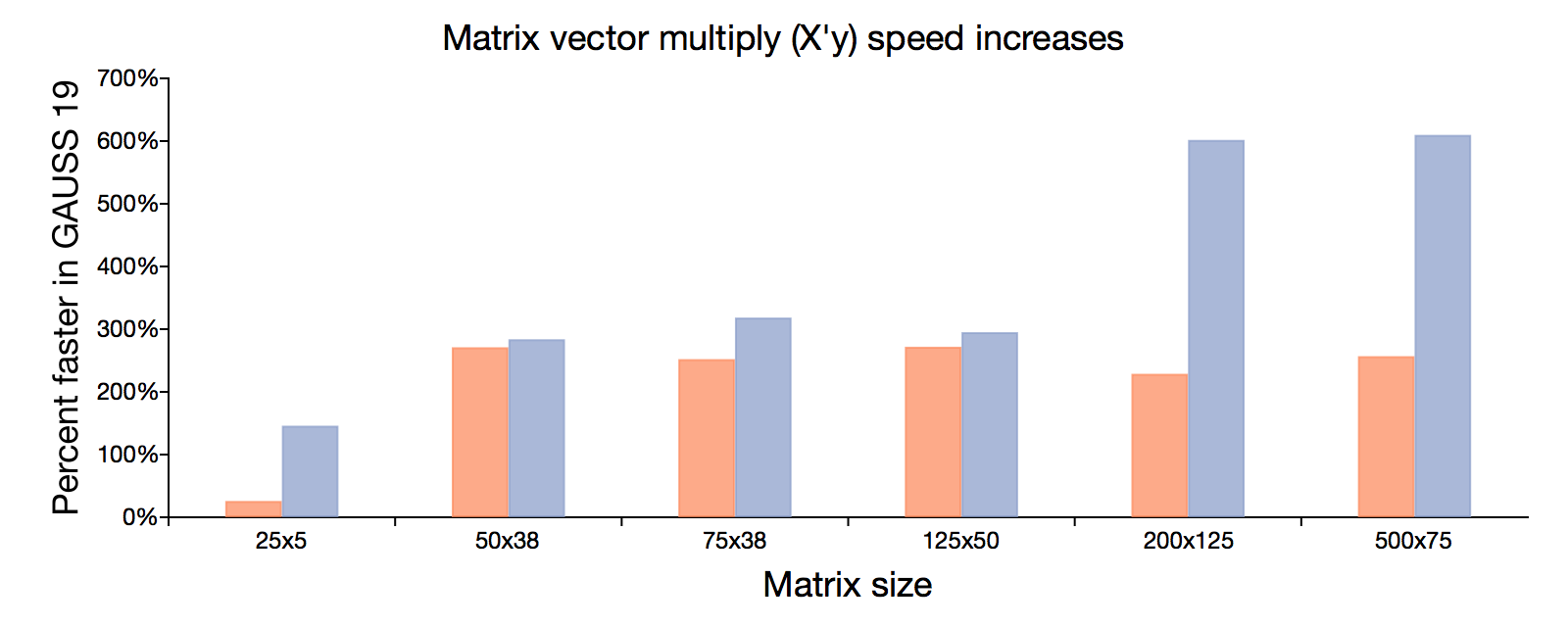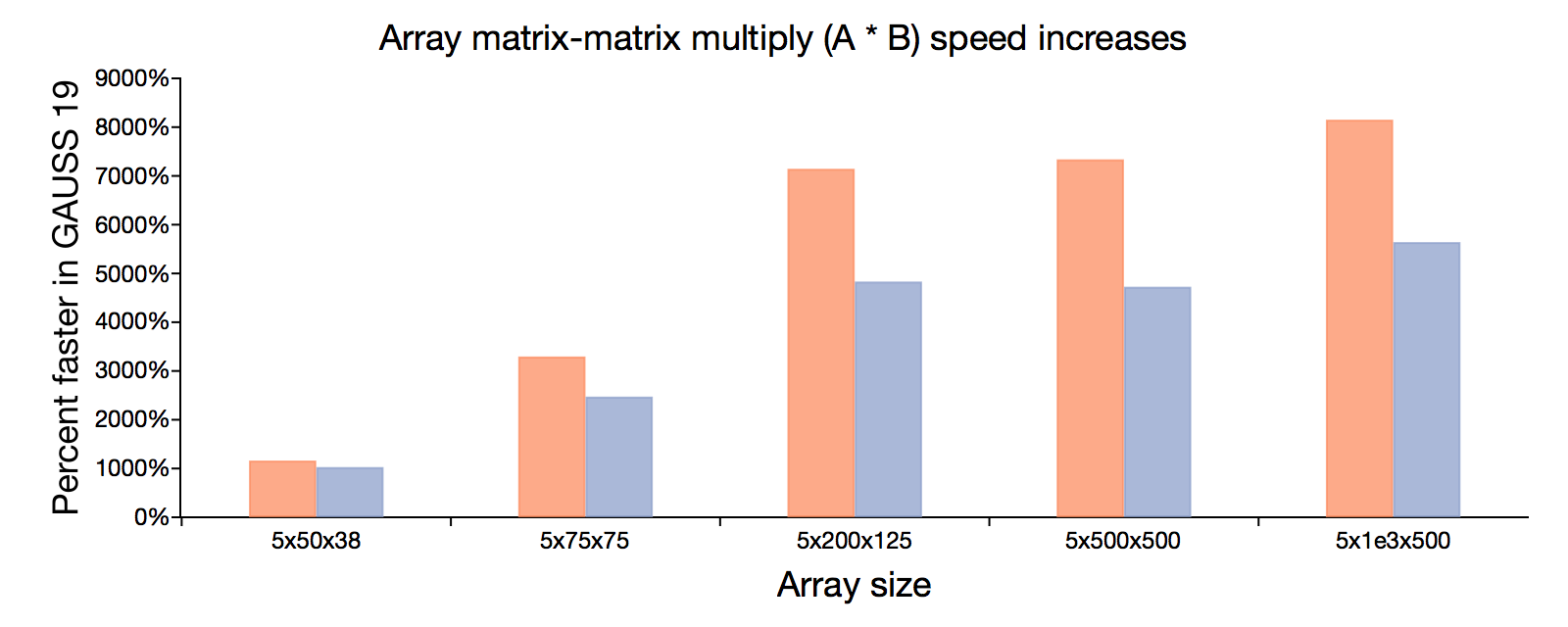# Speed improvements in GAUSS 19

## The Exponential function (exp)Higher is better. 200% faster means the computation takes 67% less time. Since speed improvements will vary based upon computer specifications, speed improvements are reported for two different computers, represented by the orange and blue bars.

## Matrix vector multiplyEach matrix is transposed and multiplied by a vector with the same number of rows as the original matrix. For example, the 125x50 matrix is transposed and then multiplied by a 125x1 vector.

## Matrix-matrix multiply of multi-dimensional arraysEach array is multiplied by another array in which the final two dimensions are switched. For example, each of the 5, 50x38 matrices in the 5x50x38 array are multiplied by the corresponding 38x50 matrices from a 5x38x50 array.